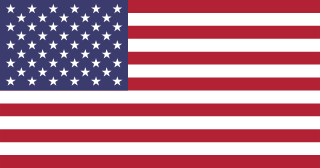close
search
How big is 30 square centimeters?
It's about three-fifths as big as a Post-it® Note (3M)
The size of a Post-it® Note (3M) is about 54 square centimeters.
(3M) (generically sticky notes, a.k.a. repositionable notes, a.k.a. repositional notes)
more_vert
It's about half as big as a Playing Card
The size of a Playing Card is about 56 square centimeters.
(ISO 216 B8 size)
more_vert
It's about four times as big as a Secure Digital (SD) card
The size of a Secure Digital (SD) card is about 7.70 square centimeters.
more_vert
It's about six-and-a-half times as big as a US QuarterThe size of a US Quarter is about 4.6220 square centimeters.
(United States quarter-dollar coin) (a.k.a. Quarter dollar, a.k.a. Washington quarter, a.k.a. two bits)
more_vert
It's about eight times as big as a Postage StampThe size of a Postage Stamp is about 4 square centimeters.
(average; United States dimensions)
more_vert
It's about eight-and-a-half times as big as a US NickelThe size of a US Nickel is about 3.5330 square centimeters.
(United States five-cent coin) (a.k.a. Jefferson nickel)
more_vert
It's about ten-and-a-half times as big as a US PennyThe size of a US Penny is about 2.850 square centimeters.
(United States one-cent coin) (a.k.a. Lincoln penny, a.k.a. Cent, a.k.a. One cent piece)
more_vert
It's about twelve times as big as a US DimeThe size of a US Dime is about 2.5190 square centimeters.
(United States ten-cent coin) (a.k.a. Roosevelt dime)
more_vert
(for 9.7 inch, Apple iPad Air MD785LL/B)
more_vert
It's about one-thirty-fifth as big as an Airplane Tray Table
The size of an Airplane Tray Table is about 1,010 square centimeters.
(average)
more_vert
(for 11-gauge wire nai)
more_vert
It's about one-three-hundred-fiftieth as big as a Bath Towel
The size of a Bath Towel is about 10,838 square centimeters.
(for Fieldcrest Luxury Solid Towels)
more_vert
It's about one-one-thousand-five-hundredth as big as a King Size Bed
The size of a King Size Bed is about 40,300 square centimeters.
(North American/Australian standard; length; mattress only)
more_vert
It's about 4,500 times as big as a Ballpoint Pen Tip
The size of a Ballpoint Pen Tip is about 0.007 square centimeters.
(a.k.a. Biro, a.k.a. ball pen, a.k.a. dot pen) (for medium tip)
more_vert
It's about one-five-thousandth as big as a Parking Space
The size of a Parking Space is about 150,000 square centimeters.
(a.k.a Parking Stall) (average; surface lot; North America)
more_vert

More Results